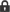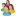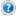### Popular Store Titles

•Tank Jacket Calculator

•Specification Sheet Collection

•PIPESIZE

•Relief Valve Sizing

•Rupture Disc Sizing

See More Titles

•Kashif Rao

what correlation to be used to correct two phase flow through orifie meter.•tarang.suthar

line size criteria for liquid and steam piping•ankur2061

Read my article at Linkedin: "Process Simulation Software - Don't be a Data Entry Operator"•Ahsan67

stack•MurtazaHakim

Exploring the subtleties of Process Design### Chemical and Process Engineering ResourcesCategory: Fluid Dynamics Question: How can I determine the choked or sonic flow in an orifice? Keywords: v1i17,choked,choke,sonic,flow,gas,orifice,calculate,determine,calculation Answer: Choked flow (also known as sonic flow) occurs when the ratio of the upstream gas pressure to the downstream gas pressure is equal to or greater than [(k + 1) / 2]k / ( k - 1) , where k is the specific heat ratio (Cp / Cv). For many gases, k ranges from about 1.1 to about 1.4, and so choked gas flow usually occurs when the upstream pressure is about twice the downstream pressure. The equation for choked flow is: Q = C A (g k d PU )1/2 [2 / (k + 1)](k +1) / (2k - 2) If the ratio of upstream to downstream gas pressure is less than [(k + 1) / 2]k / ( k - 1),then the flow is non-choked (i.e., sub-sonic) and the equation for non-choked flow is: Q = C A (2 g d PU)1/2 [k / (k - 1)]1/2 [(PD / PU)2/k - (PD / PU )(k + 1) / k ]1/2where: Q = mass flow, lb/s C = discharge coefficient (usually about 0.72) A = orifice hole area, ft2 g = 32.17 ft/s2 gravitational acceleration k = Cp / Cv = (specific heat at constant pressure) / (specific heat at constant volume) d = gas density, lb/ft3, at upstream pressure PU = upstream pressure, lbs/ft2 PD = downstream pressure, lbs/ft2

•Industrial Forum

•Student Forum

•Refining Forum

•Simulation Forum

•Relief Devices Forum

•Tank Venting Forum

### Tech Q & A Category List

•Bulk Solids

•ChE Outside the Plant

•Chemical Process Business

•Chemistry Basics

•Corrosion

•Equipment Design

•Experimentation and Testing

•Fluid Dynamics

•Heat Transfer Technology

•Industrial Utilities

•Mass Transfer

•Physical Property Information

•Plant Basics

•Plant Economics

•Preparing to Become an Engineer

•Process Control

•Reactions and Processes

•Refining

•Safety

•Separation Technology

•The Environment

•Thermodynamics